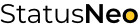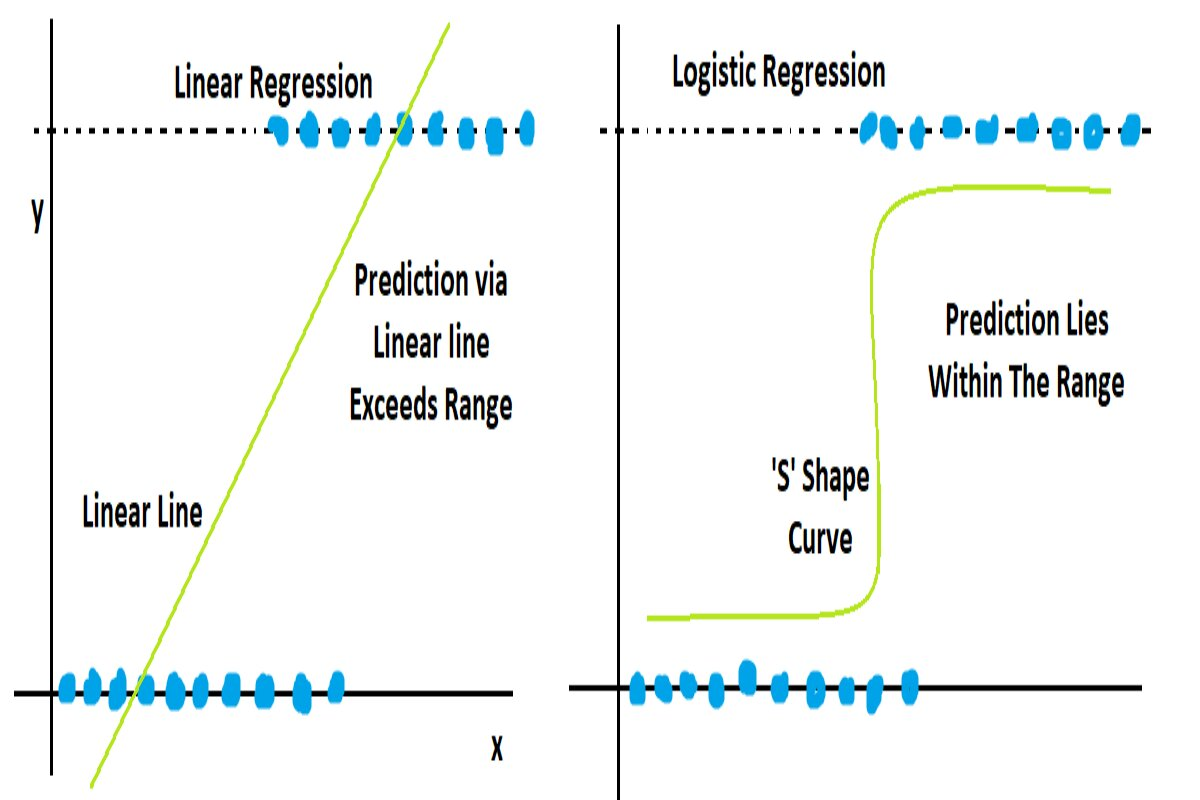WE ARE EXPERTS IN TECHNOLOGY

## Let’s Work Together# StatusNeo## Why Logistic Regression For Classification Instead Linear Regression: Let’s Get It

As we know,

Linear Regression: It is a supervised machine learning algorithm and is used for regression-related problems where the main task is predicting the continuous values. This algorithm finds the linearity between  independent variable and dependent variable. Linear regression’s prediction range is between (-∞, +∞).

Logistic Regression: It is also a type of supervised machine learning, but it can be known as a subset of linear regression. Because all the operations behind logistic regression are carried out on the basis of linear regression, But as we know, logistic regression is used for classification. Since at the time of final prediction, the logistic regression uses some log function to convert the (-∞, +∞) in 0 to 1 range.

Linear regression Find the best fit line among all the data, which will cover the maximum number of data points, and depending on this best fit line model, start to predict.

Formula :  y= m*x+c

Whether

y= dependent variable

x= independent variable

m= slope

c= intercept

In logistic regression, model draw the “S” shape sigmoid curve which travels between 0 to 1. Just because of bound (range) between 0 & 1, there is no any possibility to draw a straight line.

Formula sigmoid σ(y) = 1 / (1 + e^(-y))

Where y = m*x+c (linear regression formula)

So now formula will be: σ(y) = 1 / (1 + e^(- m*x+c))

## Now we can see some conditions by which we can conclude why not linear and why logistic

As we know, linear regression is used for continuous values, and even though we know that behind logistic regression, all operations take place on the basis of linear, still why not linear regression and why logistic?

• The first main condition of classification is range-bound, which means we need to predict between 0 and 1 only. Linear can’t predict the thing between 0 and 1, instead its range is (-∞, +∞).
• The next one is loss function. In linear regression, whatever model gets lost (errors), it comes in a large number (because operations perform on continuous values), and even if it does not, the model punishes it and increases its value just because of optimizing that loss. But in logistic regression, whatever loss is generated has a very small value. Because it’s already between 0 and 1.
• And suppose even we use linear regression for classification, while optimizing the errors derived from MSE, the model cannot get optimal updated values for parameters, and at one stage updation will stagnate. Because already classification error rates are too small, if we perform derivation with respect to MSE, the model can’t reach global minima; instead, it reaches local minima, and the problem of gradient vanishing happens.
• The next reason is data. While predicting the class (let’s assume here for binary classification), there are only two classes, and there is a possibility that whatever independent variables are there, we can find some similarities between them, so this condition violates the assumption of linear regression, which is independence; There shouldn’t be dependency between independent variables.
• The next reason is linearity. There is no possibility that we could find the linear relationship between independent and dependent variables for classification. Because in classification there are possibilities of similarity in independent variables,
• Linear regression doesn’t allow multicollinearity, but logistic regression does to a lesser extent.
• Data is divided into two classes that are not compatible for linear to perform the operation.

Now let’s see what the differences are between them.

Consultant (Digital) in StatusNeo. Master of Engineering in Data Science. Love to work on Machine Learning, NLP, Deep Learning, Transfer Learning, Computer Vision, Yolo, MlOps.